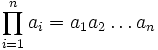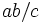# Order of a group

This article is about a basic definition in group theory. The article text may, however, contain advanced material.
VIEW: Definitions built on this | Facts about this: (facts closely related to Order of a group, all facts related to Order of a group) |Survey articles about this | Survey articles about definitions built on this
VIEW RELATED: Analogues of this | Variations of this | Opposites of this |[SHOW MORE]
This article defines an arithmetic function on groups
View other such arithmetic functions

## Definition

QUICK PHRASES: size of a group, cardinality of a group, size of the underlying set, number of elements

### Symbol-free definition

The order of a group is the cardinality (i.e., size, or number of elements) of its underlying set.

### Definition with symbols

The order of a group$G$ is the cardinality (i.e., size, or number of elements) of$G$ as a set. it is denoted as$\left| G \right|$.

Note that a finite group is a group whose underlying set is finite, i.e., the size of the underlying set is finite. The order of a finite group is thus a natural number (note that the order cannot be zero because every group contains the identity element and is hence nonempty). For an infinite group, the order is an infinite cardinal.

## Examples

• The trivial group, which is the group with only the identity element, has order$1$. In fact, it is the only group (up to isomorphism}) that has order 1.

(see the next section for more examples).

## Operations on groups and effects on the order

### Operations that admit a clear formula

Operation Input groups and their orders Output group and its order Proof and comment
external direct product of two groups$G$ has order$a$,$H$ has order$b$$G \times H$ has order$ab$ order of direct product is product of orders; the same formula works for internal direct product, which is equivalent to external direct product.
external direct product of finitely many groups$G_1, G_2, \dots, G_n$ with orders$a_1, a_2, \dots, a_n$ respectively$G_1 \times G_2 \times \dots \times G_n$ has order$\prod_{i=1}^n a_i = a_1a_2 \dots a_n$ order of direct product is product of orders; same formula works for internal direct product
external semidirect product of two groups$G$, order$a$,$H$, order$b$, acting on it$G \rtimes H$ has order$ab$ order of semidirect product is product of orders; same formula works for internal semidirect product
group extension normal subgroup$N$, order$a$, quotient group$G/N$, order$b$$ab$ follows from Lagrange's theorem
product of two subgroups$H$, order$a$,$K$ order$b$,$H \cap K$ has order$c$$ab/c$ see product formula, also second isomorphism theorem
external wreath product$H$ order$a$,$K$ with wreathing action, order$b$, acting on a set of size$n$$H \wr K$ has order$a^nb$ Note that when we take the regular action,$n = b$ and we get$a^bb$. When$K$ is the symmetric group of degree$n$ acting naturally on a set of size$n$, we get$a^nn!$.

### Operations that do not admit a clear formula

These include, for instance, measuring the sizes of homomorphism sets between two groups.

## Particular cases and general patterns

See also number of groups of given order for more information on how the number of groups of order$n$ depends on$n$.

### Small orders

Number Groups of that order More collected information on the list
1 trivial group --
2 cyclic group:Z2 --
3 cyclic group:Z3 --
4 cyclic group:Z4, Klein four-group
5 cyclic group:Z5 --
6 symmetric group:S3, cyclic group:Z6
7 cyclic group:Z7 --
8 cyclic group:Z8, direct product of Z4 and Z2, dihedral group:D8, quaternion group, elementary abelian group:E8 groups of order 8
16 (14 groups, too long to list) groups of order 16

### General facts

Nature of order What we can say about groups of that order Proof
1 unique group: trivial group
prime$p$ unique up to isomorphism: group of prime order, which is cyclic and is the additive group of a prime field equivalence of definitions of group of prime order$p^2$,$p$ prime cyclic group of prime-square order, elementary abelian group of prime-square order  ?$p^3$,$p$ prime 5 groups -- 3 abelian and 2 non-abelian
cyclicity-forcing number -- product of distinct primes such that no prime divides any other prime minus one unique cyclic group of that order classification of cyclicity-forcing numbers

## Divisibility relations

### Divisibility relations for constructed objects

Construct Divisibility statement related to order Proof Comments
subgroup order of subgroup divides order of group Lagrange's theorem Not every order dividing the order of the group is realized as the order of a subgroup, see group having subgroups of all orders dividing the group order
quotient group order of quotient group divides order of group order of quotient group divides order of group -- corollary to Lagrange's theorem
order of element in the group order of element divides order of group order of element divides order of group Follows from Lagrange's theorem because the order of an element is the order of the cyclic subgroup it generates.
set on which the group acts transitively size of such a set divides the order of the group fundamental theorem of group actions identifies any set with a transitive group action with the left coset space of a stabilizer, then we use Lagrange's theorem
conjugacy class size of conjugacy class divides order of group size of conjugacy class divides order of group in fact, it divides order of inner automorphism group and is strictly smaller for a non-abelian group
degree of irreducible representation degree of irreducible representation over a splitting field divides order of group degree of irreducible representation divides order of group in fact, degree of irreducible representation divides index of abelian normal subgroup

### Divisibility relations for other arithmetic functions

Any arithmetic function that arises as the maximum or lcm of a bunch of numbers each of which divides the order of the group, must again divide the order of the group. Some examples are given below:

Arithmetic function Description Proof that it divides order
exponent lcm of orders of all elements exponent divides order

## Analogues

An order is a size measure that works for finite groups. For infinite groups, the order, viewed as an infinite cardinal, is a very crude size measure since it is unable to differentiate between the group and subgroups of finite index. Some analogues that work are described below.

• Measure is an analogue that is used for amenable groups, and works particularly well for compact groups with a Haar measure.
• Dimension plays a role analogous to the logarithm of the order. In those situations where the orders multiply, dimensions tend to add. There are many different notions of dimension, including algebraic, analytic, and topological ones.
• For profinite groups, we can view the orders as supernatural numbers, i.e., the orders take values that are products of powers of possibly infinitely many primes. Analogues of Lagrange's and Sylow's theorems hold in these contexts.

### Order from subgroup and quotient perspectives

An infinite cyclic group (i.e., a group isomorphic to the group of integers) has no proper nontrivial finite subgroups, so from the perspective of Lagrange's theorem for subgroups, its order can be thought of as having no prime divisors. However, it has finite quotients of every order, so from the perspective of the fact that order of quotient group divides order of group, every prime divides its order.

More generally, for periodic groups and locally finite groups, the order notion must capture the possible finite subgroups that arise, whereas for residually finite groups, the order notion must capture the possible finite quotients that arise.

## Computation

The GAP command to compute the order of a group is:

Order (group);
where
group
may either be an on-the-spot definition of a group or a name for something defined earlier.